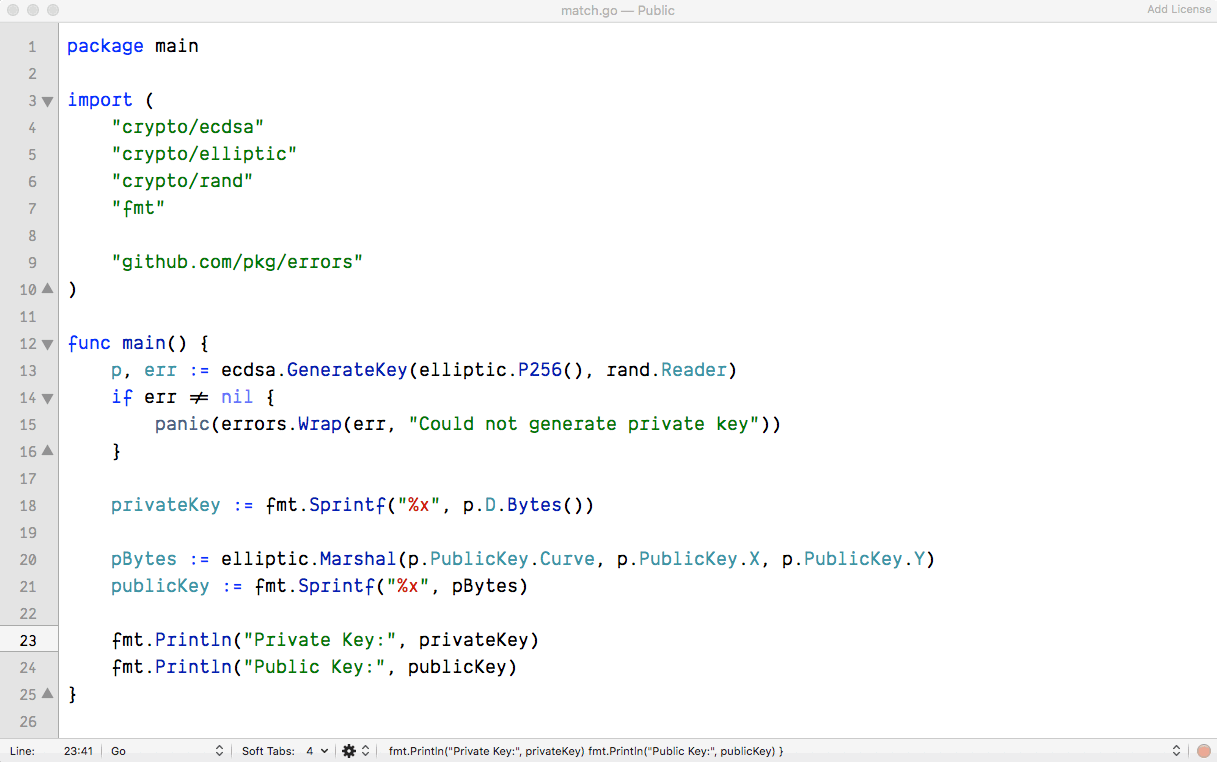Elizar Pepino

June 5, 2019

# Generating ECDH Key Pair Using Go

## What is ECDH?

Elliptic-curve Diffie–Hellman (ECDH) is an anonymous key agreement protocol that allows two parties, each having an elliptic-curve public–private key pair, to establish a shared secret over an insecure channel. This shared secret may be directly used as a key, or to derive another key. The key, or the derived key, can then be used to encrypt subsequent communications using a symmetric-key cipher. It is a variant of the Diffie–Hellman protocol using elliptic-curve cryptography.

Wiki

There’s an excellent article about Elliptic Curve Cryptography from Cloudflare’s blog. Check it out here.

## Here’s the code

``````package main

import (
"crypto/ecdsa"
"crypto/elliptic"
"crypto/rand"
"fmt"

"github.com/pkg/errors"
)

func main() {
p, err := ecdsa.GenerateKey(elliptic.P256(), rand.Reader)
if err != nil {
panic(errors.Wrap(err, "Could not generate private key"))
}

privateKey := fmt.Sprintf("%x", p.D.Bytes())

pBytes := elliptic.Marshal(p.PublicKey.Curve, p.PublicKey.X, p.PublicKey.Y)
publicKey := fmt.Sprintf("%x", pBytes)

fmt.Println("Private Key:", privateKey)
fmt.Println("Public Key:", publicKey)
}
``````

TRY IT!

Running the ☝🏽 code will print something like the following:## Let’s break the code down

### 1. Private key generation.

``````p, err := ecdsa.GenerateKey(elliptic.P256(), rand.Reader)
if err != nil {
panic(errors.Wrap(err, "Could not generate private key"))
}
``````

We generate a private key using `ecdsa.GenerateKey` function. This function takes two arguments: `elliptic.Curve` and `io.Reader` and returns an instance of `ecdsa.PrivateKey` and an `error` if there is one.

For this example, I decided to use `elliptic.P256()` for the curve and `rand.Reader` as the random reader.

Note: `elliptic.P256()` is no longer safe to use btw, but I have my reasons which I will discuss on the later part of this series.

### 2. Extract both the private and public keys’ hex values.

``````privateKey := fmt.Sprintf("%x", p.D.Bytes())
``````

The `ecdsa.PrivateKey` struct definition looks like this:

``````type PrivateKey struct {
PublicKey
D *big.Int
}
``````

So to get the readable/string value of the private key, we have to get to `D *big.Int` instance first, and then call `.Bytes()` and store the result to a hex(base16) placeholder string `"%x"`.

``````pBytes := elliptic.Marshal(p.PublicKey.Curve, p.PublicKey.X, p.PublicKey.Y)
publicKey := fmt.Sprintf("%x", pBytes)
``````

The `ecdsa.PublicKey` struct definition looks like this:

``````type PublicKey struct {
elliptic.Curve
X, Y *big.Int
}
``````

Getting the hex value of the public key needs a bit of extra work. For this example, we use `elliptic.Marshal`. There are, of course, other ways to do it, but this came to mind first while I was writing this article.

For the next part of this series, we will explore some good use cases for ECDH.

🖖🤓